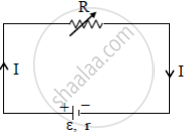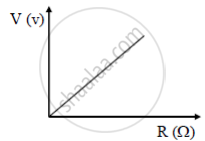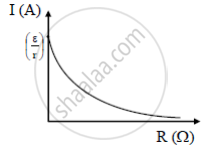# A Variable Resistor R is Connected Across a Cell of Emf ε and Internal Resistance R as Shown in the Figure. Draw a Plot Showing the Variation of - Physics

Short Note

A variable resistor R is connected across a cell of emf ε and internal resistance r as shown in the figure. Draw a plot showing the variation of
(i) Terminal voltage V and
(ii) the current I, as a function of R.#### Solution

(i) Terminal voltage across a cell as a function of R

As resistance R increases current (I) in the circuit decreases and terminal voltage (V) increases.

We know V = ε –Ir; Where ε is emf of the cell(ii)
Current I as a function of R.

The current across a cell is given by "I" = epsilon/("R"+"r")

When R increases I decreasesConcept: Temperature Dependence of Resistance
Is there an error in this question or solution?